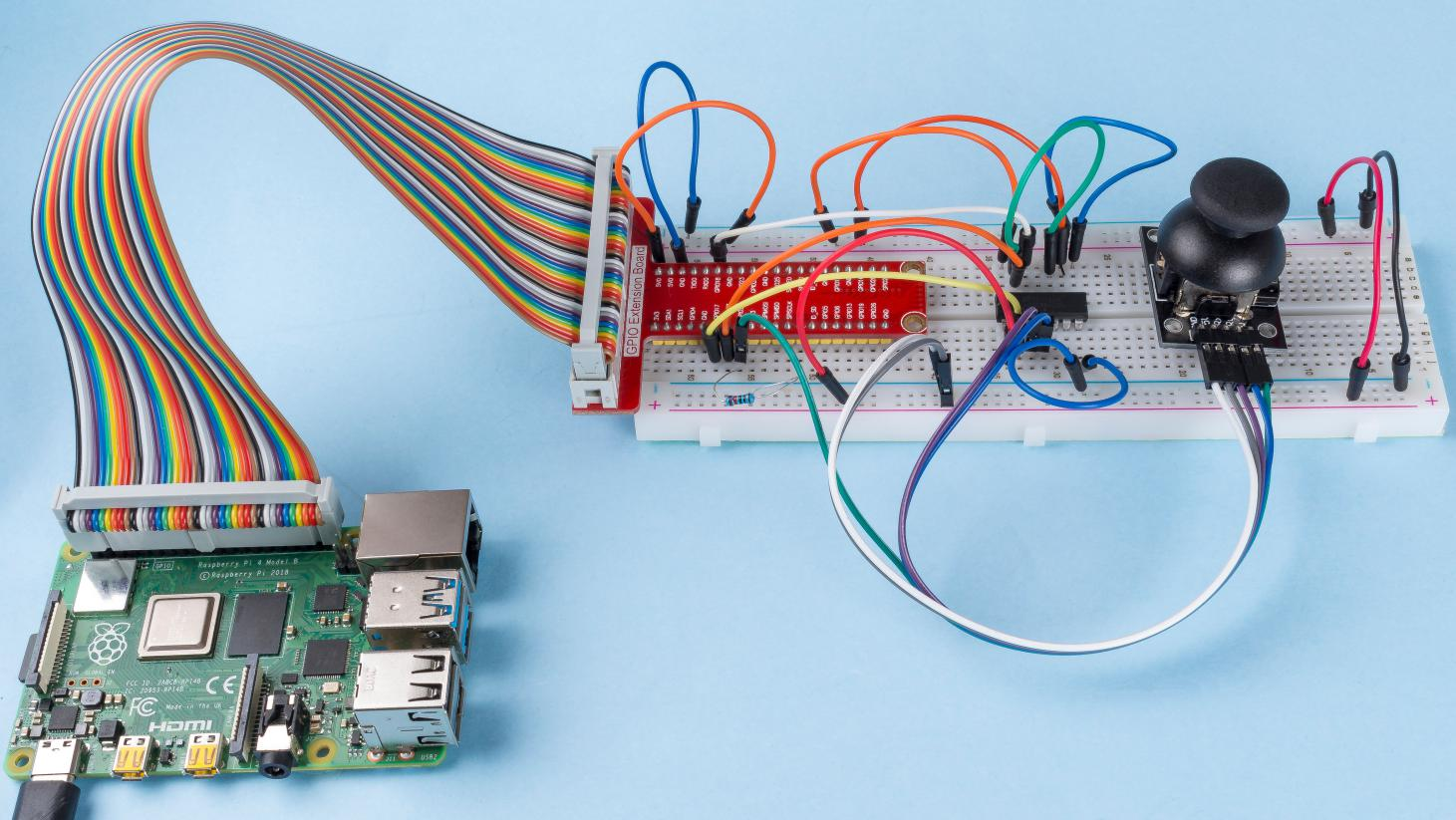# 2.1.6 Joystick¶

## Introduction¶

In this project, We’re going to learn how joystick works. We manipulate the Joystick and display the results on the screen.

## Components¶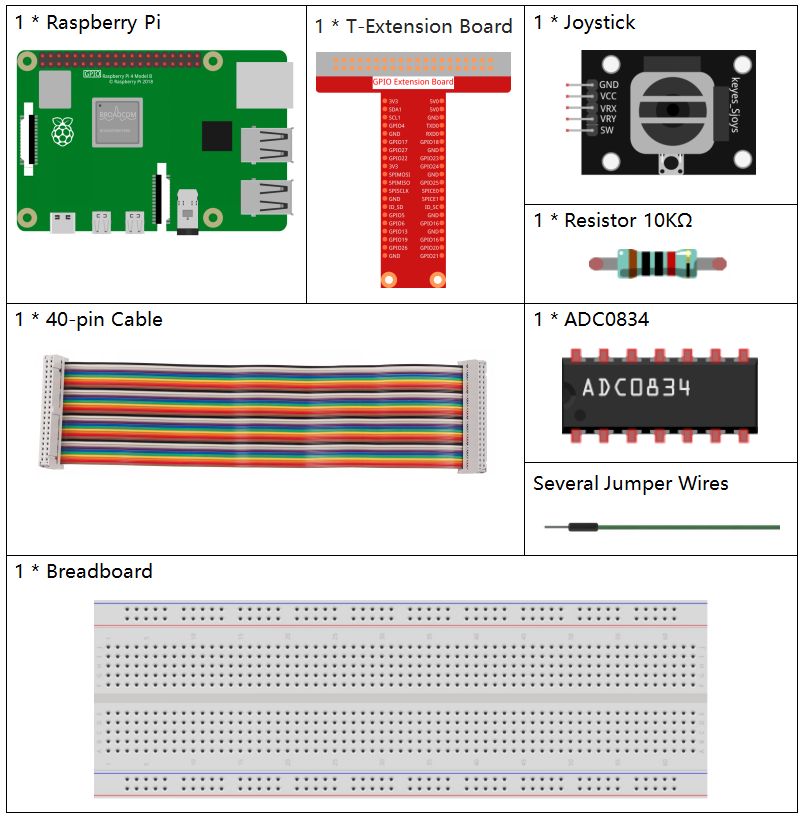## Principle¶

Joystick

The basic idea of a joystick is to translate the movement of a stick into electronic information that a computer can process.

In order to communicate a full range of motion to the computer, a joystick needs to measure the stick’s position on two axes – the X-axis (left to right) and the Y-axis (up and down). Just as in basic geometry, the X-Y coordinates pinpoint the stick’s position exactly.

To determine the location of the stick, the joystick control system simply monitors the position of each shaft. The conventional analog joystick design does this with two potentiometers, or variable resistors.

The joystick also has a digital input that is actuated when the joystick is pressed down.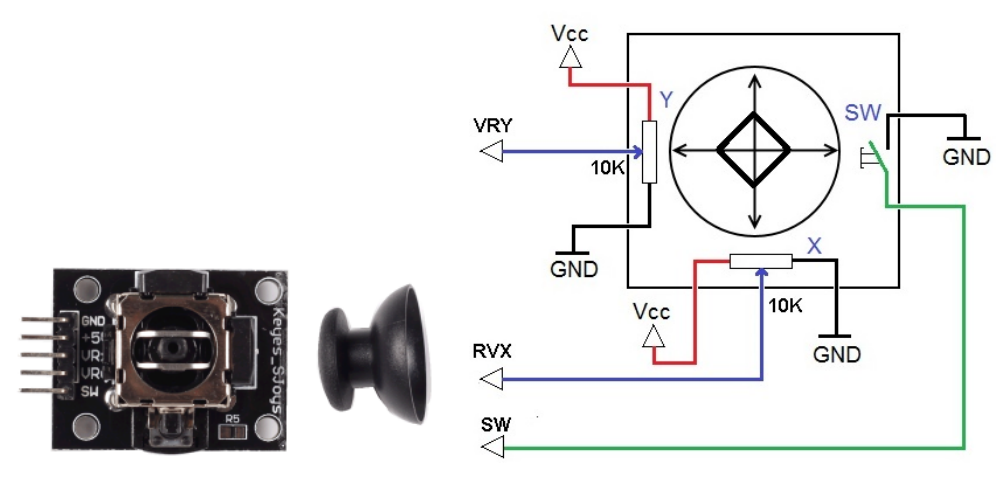## Schematic Diagram¶

When the data of joystick is read, there are some differents between axis: data of X and Y axis is analog, which need to use ADC0834 to convert the analog value to digital value. Data of Z axis is digital, so you can directly use the GPIO to read, or you can also use ADC to read.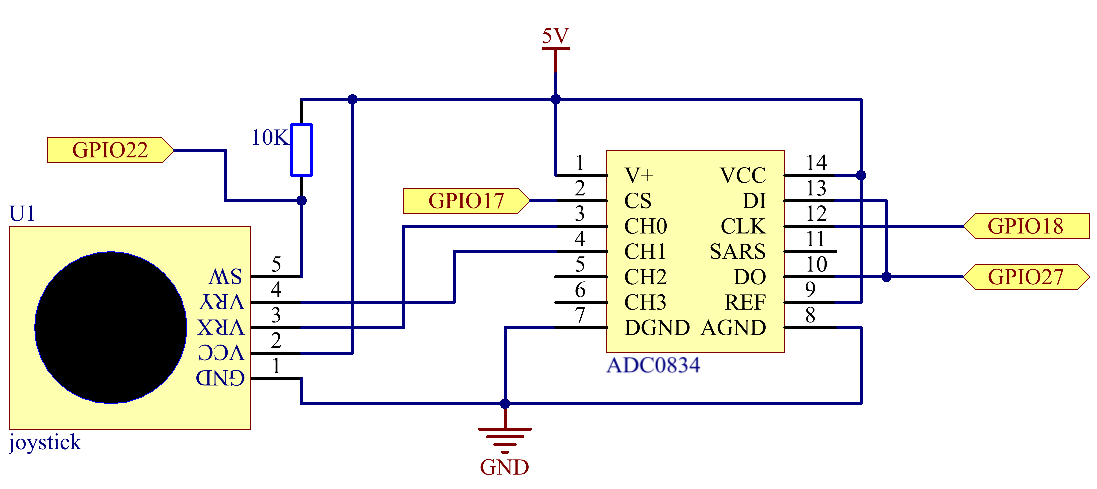## Experimental Procedures¶

Step 1: Build the circuit.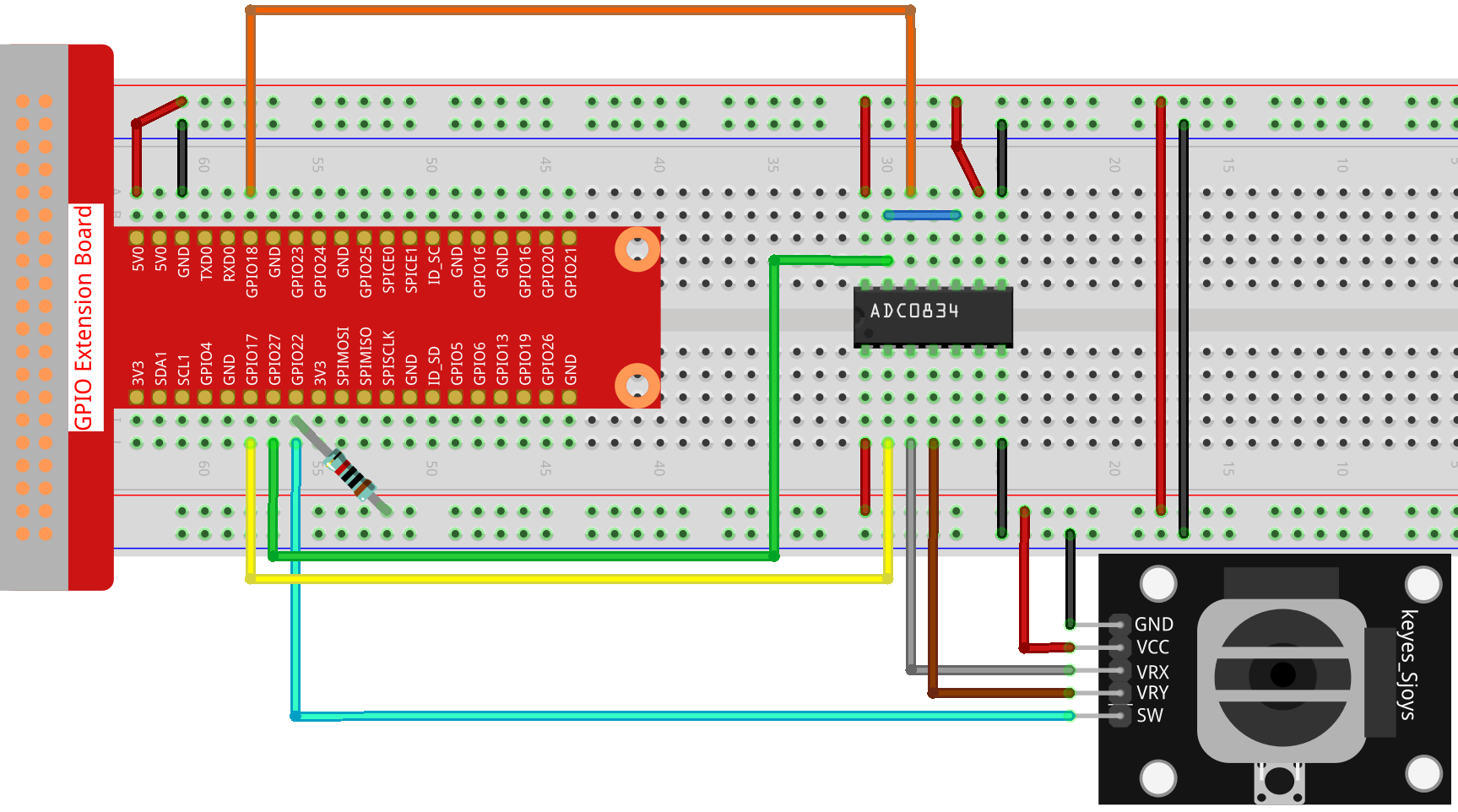### For C Language Users¶

Step 2: Go to the folder of the code.

```cd /home/pi/davinci-kit-for-raspberry-pi/c/2.1.6/
```

Step 3: Compile the code.

```gcc 2.1.6_Joystick.c -lwiringPi
```

Step 4: Run the executable file.

```sudo ./a.out
```

After the code runs, turn the Joystick, then the corresponding values of x, y, Btn are displayed on screen.

Note

If it does not work after running, or there is an error prompt: “wiringPi.h: No such file or directory”, please refer to C code is not working?.

Code

```#include <wiringPi.h>
#include <stdio.h>
#include <softPwm.h>

typedef unsigned char uchar;
typedef unsigned int uint;

#define     BtnPin    3

{
uchar i;
uchar dat1=0, dat2=0;
int sel = channel > 1 & 1;
int odd = channel & 1;
// Start bit
//Single End mode
// ODD
//Select
for(i=0;i<8;i++)
{
}
for(i=0;i<8;i++)
{
}
return(dat1==dat2) ? dat1 : 0;
}
int main(void)
{
uchar x_val;
uchar y_val;
uchar btn_val;
if(wiringPiSetup() == -1){ //when initialize wiring failed,print messageto screen
printf("setup wiringPi failed !");
return 1;
}
pinMode(BtnPin,  INPUT);
pullUpDnControl(BtnPin, PUD_UP);

while(1){
printf("x = %d, y = %d, btn = %d\n", x_val, y_val, btn_val);
delay(100);
}
return 0;
}
```

Code Explanation

```uchar get_ADC_Result(uint channel)
{
uchar i;
uchar dat1=0, dat2=0;
int sel = channel > 1 & 1;
int odd = channel & 1;
// Start bit
//Single End mode
......
```

The working process of the function is detailed in 2.1.4 Potentiometer.

```while(1){
printf("x = %d, y = %d, btn = %d\n", x_val, y_val, btn_val);
delay(100);
}
```

VRX and VRY of Joystick are connected to CH0, CH1 of ADC0834 respectively. So the function getResult() is called to read the values of CH0 and CH1. Then the read values should be stored in the variables x_val and y_val. In addition, read the value of SW of joystick and store it into the variable Btn_val. Finally, the values of x_val, y_val and Btn_val shall be printed with print() function.

### For Python Language Users¶

Step 2: Go to the folder of the code.

```cd /home/pi/davinci-kit-for-raspberry-pi/python/
```

Step 3: Run.

```sudo python3 2.1.6_Joystick.py
```

After the code runs, turn the Joystick, then the corresponding values of x, y, Btn are displayed on screen.

Code

Note

You can Modify/Reset/Copy/Run/Stop the code below. But before that, you need to go to source code path like `davinci-kit-for-raspberry-pi/python`.

```import RPi.GPIO as GPIO
import time

BtnPin = 22

def setup():
# Set the GPIO modes to BCM Numbering
GPIO.setmode(GPIO.BCM)
GPIO.setup(BtnPin, GPIO.IN, pull_up_down=GPIO.PUD_UP)

def destroy():
# Release resource
GPIO.cleanup()

def loop():
while True:
Btn_val = GPIO.input(BtnPin)
print ('X: %d  Y: %d  Btn: %d' % (x_val, y_val, Btn_val))
time.sleep(0.2)

if __name__ == '__main__':
setup()
try:
loop()
except KeyboardInterrupt: # When 'Ctrl+C' is pressed, the program destroy() will be executed.
destroy()
```

Code Explanation

```def loop():
while True: